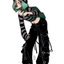MathematicsOpenStudy (anonymous):

how do you find the circumferenceOpenStudy (ragingsquirrel):

of a circle?OpenStudy (anonymous):

yesOpenStudy (ragingsquirrel):

multiply the diameter by Pi. (3.14)OpenStudy (anonymous):

you think i tryedOpenStudy (ragingsquirrel):

so do you know the diameter or the radius?OpenStudy (anonymous):

like it says 15 cm so i times by pi (3.14) rightOpenStudy (aravindg):

2*pi*rOpenStudy (ragingsquirrel):

you had to post that 4 times...OpenStudy (anonymous):

circumferrence of a circle =2*3.14*r where r is the radius of the circleOpenStudy (anonymous):

i ask a yes or no ?OpenStudy (anonymous):

yesOpenStudy (ragingsquirrel):

Oops i mean diameter.OpenStudy (ragingsquirrel):

But yes. Just multiply 15 by 3.14.OpenStudy (anonymous):

thankss talk latterOpenStudy (ragingsquirrel):OpenStudy (amistre64):

2r = d 2pi r = pi dOpenStudy (anonymous):

thanksOpenStudy (anonymous):

$2\pi*r$OpenStudy (ragingsquirrel):

;)OpenStudy (anonymous):

P (circunference)=2pi*r

Latest Questionshayhay6: Which of the following shows the correct steps to find the value of 8 ^1/3 Option
16 seconds ago 0 Replies 0 Medalsshalong: What was John Calhounu2019s major objection to high tariffs?
52 minutes ago 0 Replies 0 Medalshayhay6: Give an example of an equation of a line with an undefined slope. What type of li
1 hour ago 1 Reply 0 Medalshayhay6: What is the equation of a line that passes through the point (5, -3) and has a sl
1 hour ago 1 Reply 0 Medalspiperkoebel: Does anyone know the coordinates of two points on the line y = u22122?
1 hour ago 1 Reply 0 Medalslolokrat: anyone good with microeconomics that can help me find out where i went wrong on m
12 hours ago 0 Replies 0 MedalsAsleepAndUnafraid21: URGENT PLZ HELP NOW Part C: Find the distance from B to E and from P to E. Show your work.
14 hours ago 49 Replies 5 MedalsKachirikutoka: How many solutions does the following equation have? |3x + 12| = 18 a No solution
18 hours ago 1 Reply 1 MedalAsleepAndUnafraid21: In the figure below, segment DE is parallel to segment BC and segment EF is parallel to AB: The figure shows triangle ABC with segments DE and DF.
15 hours ago 11 Replies 0 MedalsAsleepAndUnafraid21: In the figure below, segment DE is parallel to segment BC and segment EF is parallel to AB: The figure shows triangle ABC with segments DE and DF.
19 hours ago 1 Reply 0 Medals